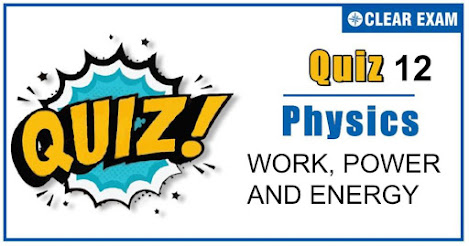## [LATEST]\$type=sticky\$show=home\$rm=0\$va=0\$count=4\$va=0

As per analysis for previous years, it has been observed that students preparing for NEET find Physics out of all the sections to be complex to handle and the majority of them are not able to comprehend the reason behind it. This problem arises especially because these aspirants appearing for the examination are more inclined to have a keen interest in Biology due to their medical background. Furthermore, sections such as Physics are dominantly based on theories, laws, numerical in comparison to a section of Biology which is more of fact-based, life sciences, and includes substantial explanations. By using the table given below, you easily and directly access to the topics and respective links of MCQs. Moreover, to make learning smooth and efficient, all the questions come with their supportive solutions to make utilization of time even more productive. Students will be covered for all their studies as the topics are available from basics to even the most advanced. .

Q1. The block of mass M moving on the frictionless horizontal surface collides with the spring of spring constant k and compresses it by length L. The maximum momentum of the block after collides is?
•  √MkL
•  (kL2)/2M
•  Zero
•  (ML2)/k
Solution
Momentum would be maximum when KE would be maximum and this is the case when total elastic PE is converted KE. According to conservation of energy 1/2 kL2=1/2 Mv2 Or kL2=(Mv)2/M MKL2=p2 (p=Mv) ∴p=L√MK

Q2. In which case does the potential energy decrease?
•  On compressing a spring
•  On stretching a spring
•  On moving a body against gravitational force
•  On the rising of an air bubble in water
Solution
In compression or extension of a spring work is done against restoring force In moving a body against gravity work is done against gravitational force of attraction It means in all three cases potential energy of the system increases But when the bubble rises in the direction of upthrust force then system works so the potential energy of the system decreases

Q3. Two bodies A and B have masses 2 kg and 5 kg respectively. Each one is acted upon by a force of 4 kg wt. If they acquire the same kinetic energy in times tA and tB, then the ratio tA/tB is ?
•  1/2
•  2
•  2/5
•  5/6
Solution
According to question, 1/2 mA vA2=1/2 mB vB2 ⇒vA/vB =√(mB/mA )=√(5/20)=1/2 Using Impulse Momentum (F∆tA)/(F∆tB )=(mA ∆vA)/(mB ∆vB )⇒(∆tA)/(∆tB )=20/5×1/2=2

Q4. If a lighter body (Mass M1 and velocity V1) and a heavier body (mass M2 and velocity V2) have the same kinetic energy, then?
•  M2 V2less than M1 V1
•  M2 V2=M1 V1
•  M2 V1=M1 V2
•  M2 V2>M1 V1
Solution
P=√2ME. If kinetic energy are equal then P∝√m i.e., heavier body posses large momentum As M1less than M2 therefore M1 V1less than M2 V2

Q5. A body moves a distance of 10 m along a straight line under action of 5 N force. If work done is 25 J, then angle between the force and direction of motion of the body will be?
•  750
•  600
•  450
•  300
Solution
Given W=25 J,F=5 N,∆s=10m Work=Force×displacement W=(F cos⁡θ)×∆s Or cos⁡θ=W/(F∙∆s) Or cos⁡θ=25/(5×10)=1/2 or θ=cos-1)⁡(1/2)=60° Hence, angle between force and direction of body is 60°.

Q6. A body of mass 5 kg moving with a velocity 10 m/s collides with another body of the mass 20 kg at, rest and comes to rest. The velocity of the second body due to collision is?
•  2.5 m/s
•  5 m/s
•  7.5 m/s
•  10 m/s
Solution
Momentum conservation 5×10+20×0=5×0+20×v⇒v=2.5 m/s

Q7. An object of mass m is tied to a string of length L and a variable horizontal force is applied on it which starts at zero and gradually increases until the string makes an angel θ with the vertical. Work done by the force F is?
•  mgL(1-sin⁡θ)
•  mgL
•  mgL(1-cos⁡θ)
•  mgL(1+cos⁡θ)
Solution
W=∆K or WT+Wg+WF=0 (Since, change in kinetic energy is zero)
Here, WT= work done by tension = 0 Wg= work done by fore of gravity =-mgh =-mgL(1-cos⁡θ) ∴ WF=-Wg=mgL(1-cos⁡θ)

Q8. A spring with spring constant k is extended from x=0to x=x1. The work done will be?
•  kx12
•  1/2 kx12
•  2kx12
•  2kx1
Solution
1/2 kx12

Q9. A ball of mass m falls vertically to the ground from a height h1 and rebound to a height h2. The change in momentum of the ball on striking the ground is?
•  mg(h1-h2)
•  mg(√(2gh1 )+√(2gh2 ))
•  m√(2g(h1+h2))
•  m√2g(h1+h2)
Solution
When ball falls vertically downward from height h1its velocity v1=√(2gh1 ) And its velocity after collision v2=√(2gh2 ) Change in momentum ∆P=m(v2-v1 )=m(√(2gh1 )+√(2gh2 )) [Because v1and v2 are opposite in direction]

Q10. A body of mass M1 collides elastically with another mass M2 at rest. There is maximum transfer of energy when?:
•  M1>M2
•  M1less than M2
•  M1=M2
•  Same for all values of M1 and M2
Solution
M1=M2## Want to know more

Please fill in the details below:

## Latest NEET Articles\$type=three\$c=3\$author=hide\$comment=hide\$rm=hide\$date=hide\$snippet=hide

Name

ltr
item
BEST NEET COACHING CENTER | BEST IIT JEE COACHING INSTITUTE | BEST NEET & IIT JEE COACHING: Work,Power and Energy Quiz 12
Work,Power and Energy Quiz 12
https://1.bp.blogspot.com/-6yGjbLV0qjM/X6Djea6AnyI/AAAAAAAACm4/fFqrFD3Mook0nvsSipXKL-IsnxrjDc8QgCLcBGAsYHQ/w469-h246/Quiz%2BImage%2BTemplate%2B%252811%2529%2B%25282%2529.jpg
https://1.bp.blogspot.com/-6yGjbLV0qjM/X6Djea6AnyI/AAAAAAAACm4/fFqrFD3Mook0nvsSipXKL-IsnxrjDc8QgCLcBGAsYHQ/s72-w469-c-h246/Quiz%2BImage%2BTemplate%2B%252811%2529%2B%25282%2529.jpg
BEST NEET COACHING CENTER | BEST IIT JEE COACHING INSTITUTE | BEST NEET & IIT JEE COACHING
https://www.cleariitmedical.com/2020/11/WorkPowerEnergyQuiz12.html
https://www.cleariitmedical.com/
https://www.cleariitmedical.com/
https://www.cleariitmedical.com/2020/11/WorkPowerEnergyQuiz12.html
true
7783647550433378923
UTF-8

STAY CONNECTED